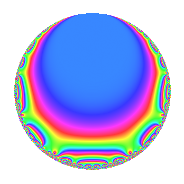# Properties

 Label 4025.2.a.iLevel 4025 Weight 2 Character orbit 4025.a Self dual Yes Analytic conductor 32.140 Analytic rank 1 Dimension 2 CM No Inner twists 1

# Related objects

## Newspace parameters

 Level: $$N$$ = $$4025 = 5^{2} \cdot 7 \cdot 23$$ Weight: $$k$$ = $$2$$ Character orbit: $$[\chi]$$ = 4025.a (trivial)

## Newform invariants

 Self dual: Yes Analytic conductor: $$32.1397868136$$ Analytic rank: $$1$$ Dimension: $$2$$ Coefficient field: $$\Q(\sqrt{5})$$ Coefficient ring: $$\Z[a_1, a_2]$$ Coefficient ring index: $$1$$ Fricke sign: $$1$$ Sato-Tate group: $\mathrm{SU}(2)$

## $q$-expansion

Coefficients of the $$q$$-expansion are expressed in terms of $$\beta = \frac{1}{2}(1 + \sqrt{5})$$. We also show the integral $$q$$-expansion of the trace form.

 $$f(q)$$ $$=$$ $$q$$ $$+ \beta q^{2}$$ $$+ q^{3}$$ $$+ ( -1 + \beta ) q^{4}$$ $$+ \beta q^{6}$$ $$+ q^{7}$$ $$+ ( 1 - 2 \beta ) q^{8}$$ $$-2 q^{9}$$ $$+O(q^{10})$$ $$q$$ $$+ \beta q^{2}$$ $$+ q^{3}$$ $$+ ( -1 + \beta ) q^{4}$$ $$+ \beta q^{6}$$ $$+ q^{7}$$ $$+ ( 1 - 2 \beta ) q^{8}$$ $$-2 q^{9}$$ $$+ ( 2 - 4 \beta ) q^{11}$$ $$+ ( -1 + \beta ) q^{12}$$ $$+ ( 1 + 2 \beta ) q^{13}$$ $$+ \beta q^{14}$$ $$-3 \beta q^{16}$$ $$-2 \beta q^{18}$$ $$+ ( -6 + 2 \beta ) q^{19}$$ $$+ q^{21}$$ $$+ ( -4 - 2 \beta ) q^{22}$$ $$+ q^{23}$$ $$+ ( 1 - 2 \beta ) q^{24}$$ $$+ ( 2 + 3 \beta ) q^{26}$$ $$-5 q^{27}$$ $$+ ( -1 + \beta ) q^{28}$$ $$+ ( 1 + 4 \beta ) q^{29}$$ $$-9 q^{31}$$ $$+ ( -5 + \beta ) q^{32}$$ $$+ ( 2 - 4 \beta ) q^{33}$$ $$+ ( 2 - 2 \beta ) q^{36}$$ $$+ ( 2 - 6 \beta ) q^{37}$$ $$+ ( 2 - 4 \beta ) q^{38}$$ $$+ ( 1 + 2 \beta ) q^{39}$$ $$+ ( -1 + 2 \beta ) q^{41}$$ $$+ \beta q^{42}$$ $$+ 4 \beta q^{43}$$ $$+ ( -6 + 2 \beta ) q^{44}$$ $$+ \beta q^{46}$$ $$+ ( 1 - 4 \beta ) q^{47}$$ $$-3 \beta q^{48}$$ $$+ q^{49}$$ $$+ ( 1 + \beta ) q^{52}$$ $$+ ( -10 + 2 \beta ) q^{53}$$ $$-5 \beta q^{54}$$ $$+ ( 1 - 2 \beta ) q^{56}$$ $$+ ( -6 + 2 \beta ) q^{57}$$ $$+ ( 4 + 5 \beta ) q^{58}$$ $$+ ( -4 - 4 \beta ) q^{59}$$ $$+ ( 6 - 12 \beta ) q^{61}$$ $$-9 \beta q^{62}$$ $$-2 q^{63}$$ $$+ ( 1 + 2 \beta ) q^{64}$$ $$+ ( -4 - 2 \beta ) q^{66}$$ $$+ ( 6 - 10 \beta ) q^{67}$$ $$+ q^{69}$$ $$+ ( -9 + 2 \beta ) q^{71}$$ $$+ ( -2 + 4 \beta ) q^{72}$$ $$+ ( 3 - 6 \beta ) q^{73}$$ $$+ ( -6 - 4 \beta ) q^{74}$$ $$+ ( 8 - 6 \beta ) q^{76}$$ $$+ ( 2 - 4 \beta ) q^{77}$$ $$+ ( 2 + 3 \beta ) q^{78}$$ $$+ ( -6 + 2 \beta ) q^{79}$$ $$+ q^{81}$$ $$+ ( 2 + \beta ) q^{82}$$ $$+ ( -4 + 4 \beta ) q^{83}$$ $$+ ( -1 + \beta ) q^{84}$$ $$+ ( 4 + 4 \beta ) q^{86}$$ $$+ ( 1 + 4 \beta ) q^{87}$$ $$+ 10 q^{88}$$ $$+ ( 4 - 8 \beta ) q^{89}$$ $$+ ( 1 + 2 \beta ) q^{91}$$ $$+ ( -1 + \beta ) q^{92}$$ $$-9 q^{93}$$ $$+ ( -4 - 3 \beta ) q^{94}$$ $$+ ( -5 + \beta ) q^{96}$$ $$+ 6 \beta q^{97}$$ $$+ \beta q^{98}$$ $$+ ( -4 + 8 \beta ) q^{99}$$ $$+O(q^{100})$$ $$\operatorname{Tr}(f)(q)$$ $$=$$ $$2q$$ $$\mathstrut +\mathstrut q^{2}$$ $$\mathstrut +\mathstrut 2q^{3}$$ $$\mathstrut -\mathstrut q^{4}$$ $$\mathstrut +\mathstrut q^{6}$$ $$\mathstrut +\mathstrut 2q^{7}$$ $$\mathstrut -\mathstrut 4q^{9}$$ $$\mathstrut +\mathstrut O(q^{10})$$ $$2q$$ $$\mathstrut +\mathstrut q^{2}$$ $$\mathstrut +\mathstrut 2q^{3}$$ $$\mathstrut -\mathstrut q^{4}$$ $$\mathstrut +\mathstrut q^{6}$$ $$\mathstrut +\mathstrut 2q^{7}$$ $$\mathstrut -\mathstrut 4q^{9}$$ $$\mathstrut -\mathstrut q^{12}$$ $$\mathstrut +\mathstrut 4q^{13}$$ $$\mathstrut +\mathstrut q^{14}$$ $$\mathstrut -\mathstrut 3q^{16}$$ $$\mathstrut -\mathstrut 2q^{18}$$ $$\mathstrut -\mathstrut 10q^{19}$$ $$\mathstrut +\mathstrut 2q^{21}$$ $$\mathstrut -\mathstrut 10q^{22}$$ $$\mathstrut +\mathstrut 2q^{23}$$ $$\mathstrut +\mathstrut 7q^{26}$$ $$\mathstrut -\mathstrut 10q^{27}$$ $$\mathstrut -\mathstrut q^{28}$$ $$\mathstrut +\mathstrut 6q^{29}$$ $$\mathstrut -\mathstrut 18q^{31}$$ $$\mathstrut -\mathstrut 9q^{32}$$ $$\mathstrut +\mathstrut 2q^{36}$$ $$\mathstrut -\mathstrut 2q^{37}$$ $$\mathstrut +\mathstrut 4q^{39}$$ $$\mathstrut +\mathstrut q^{42}$$ $$\mathstrut +\mathstrut 4q^{43}$$ $$\mathstrut -\mathstrut 10q^{44}$$ $$\mathstrut +\mathstrut q^{46}$$ $$\mathstrut -\mathstrut 2q^{47}$$ $$\mathstrut -\mathstrut 3q^{48}$$ $$\mathstrut +\mathstrut 2q^{49}$$ $$\mathstrut +\mathstrut 3q^{52}$$ $$\mathstrut -\mathstrut 18q^{53}$$ $$\mathstrut -\mathstrut 5q^{54}$$ $$\mathstrut -\mathstrut 10q^{57}$$ $$\mathstrut +\mathstrut 13q^{58}$$ $$\mathstrut -\mathstrut 12q^{59}$$ $$\mathstrut -\mathstrut 9q^{62}$$ $$\mathstrut -\mathstrut 4q^{63}$$ $$\mathstrut +\mathstrut 4q^{64}$$ $$\mathstrut -\mathstrut 10q^{66}$$ $$\mathstrut +\mathstrut 2q^{67}$$ $$\mathstrut +\mathstrut 2q^{69}$$ $$\mathstrut -\mathstrut 16q^{71}$$ $$\mathstrut -\mathstrut 16q^{74}$$ $$\mathstrut +\mathstrut 10q^{76}$$ $$\mathstrut +\mathstrut 7q^{78}$$ $$\mathstrut -\mathstrut 10q^{79}$$ $$\mathstrut +\mathstrut 2q^{81}$$ $$\mathstrut +\mathstrut 5q^{82}$$ $$\mathstrut -\mathstrut 4q^{83}$$ $$\mathstrut -\mathstrut q^{84}$$ $$\mathstrut +\mathstrut 12q^{86}$$ $$\mathstrut +\mathstrut 6q^{87}$$ $$\mathstrut +\mathstrut 20q^{88}$$ $$\mathstrut +\mathstrut 4q^{91}$$ $$\mathstrut -\mathstrut q^{92}$$ $$\mathstrut -\mathstrut 18q^{93}$$ $$\mathstrut -\mathstrut 11q^{94}$$ $$\mathstrut -\mathstrut 9q^{96}$$ $$\mathstrut +\mathstrut 6q^{97}$$ $$\mathstrut +\mathstrut q^{98}$$ $$\mathstrut +\mathstrut O(q^{100})$$

## Embeddings

For each embedding $$\iota_m$$ of the coefficient field, the values $$\iota_m(a_n)$$ are shown below.

For more information on an embedded modular form you can click on its label.

Label $$\iota_m(\nu)$$ $$a_{2}$$ $$a_{3}$$ $$a_{4}$$ $$a_{5}$$ $$a_{6}$$ $$a_{7}$$ $$a_{8}$$ $$a_{9}$$ $$a_{10}$$
1.1
 −0.618034 1.61803
−0.618034 1.00000 −1.61803 0 −0.618034 1.00000 2.23607 −2.00000 0
1.2 1.61803 1.00000 0.618034 0 1.61803 1.00000 −2.23607 −2.00000 0
 $$n$$: e.g. 2-40 or 990-1000 Significant digits: Format: Complex embeddings Normalized embeddings Satake parameters Satake angles

## Inner twists

This newform does not admit any (nontrivial) inner twists.

## Atkin-Lehner signs

$$p$$ Sign
$$5$$ $$1$$
$$7$$ $$-1$$
$$23$$ $$-1$$

## Hecke kernels

This newform can be constructed as the intersection of the kernels of the following linear operators acting on $$S_{2}^{\mathrm{new}}(\Gamma_0(4025))$$:

 $$T_{2}^{2}$$ $$\mathstrut -\mathstrut T_{2}$$ $$\mathstrut -\mathstrut 1$$ $$T_{3}$$ $$\mathstrut -\mathstrut 1$$ $$T_{11}^{2}$$ $$\mathstrut -\mathstrut 20$$